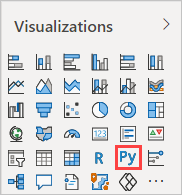top of page
Search
• Alibek Jakupov

# Power BI : Partially Colorize Line ChartsPower BI is an interactive data visualization software product developed by Microsoft with a primary focus on business intelligence.It is part of the Microsoft Power Platform. Power BI is a collection of software services, apps, and connectors that work together to turn unrelated sources of data into coherent, visually immersive, and interactive insights. Data may be input by reading directly from a database, webpage, or structured files such as spreadsheets, CSV, XML, and JSON.

If you have already faced the issue of partially colorizing your line charts, then you should be already quite familiar with this tool, thus we will directly switch to the problem.

Imagine the following scenario: you are requested to plot two time series, and compare them to each other. The comparison consists in colorizing the parts where the charts vary, thus allowing the final user instantly see the similarities between two graphs. The user should also be able to control the similarity level directly from the dashboard.

Seems like a custom line chart, right? But after looking through the Power BI visuals store, I didn't find anything satisfying my needs, so I created a simple Python visual. Hopefully this will be useful for some of the readers. Up we go!

## Data Format

Any time series data, with a timestamp and a value column, is suitable for our use case. There may also be an index instead of a timestamp column. It is also required to fusion the two time series into one single dataset, so you will need an identifier for your time series. Your final dataset should look something like this## Create Power BI Parameter

This step is quite straightforward, we need simply to allow our users to control the similarity level. Here's the DAX formula:

`Similarity Parameter = GENERATESERIES(0, 2000, 0.1)`

Then, when you add this parameter to the visual it will add a new column in your dataset, which will allow you to control the similarity level.## Create a Python visual

Select the Python visual icon in the Power BI Desktop Visualizations pane.In the Enable script visuals dialog box that appears, select Enable. A placeholder Python visual image appears on the report canvas, and the Python script editor appears along the bottom of the center pane. Drag your dataset and Similarity Parameter fields to the Values section where it says Add data fields here.

Based on your selections, the Python script editor generates the following binding code.

• The editor creates a dataset dataframe with the fields you add.

• The default aggregation is Don't summarize.

• Similar to table visuals, fields are grouped and duplicate rows appear only once.

With the dataframe automatically generated by the fields you selected, you can write a Python script that results in plotting to the Python default device. When the script is complete, select the Run icon from the Python script editor title bar to run the script and generate the visual.

## Write Some Code

Create the similarity calculation function

```def calculate_difference(row):
differences = [abs(j-i) for i, j in zip(row[:-1], row[1:])]
if len(differences) == 0:
output = None
else:
output = sum(differences)/len(differences)
return output```

Obtain the similarity parameter

`SIMILARITY_RATE = dataset['Similarity Parameter Value']`

Transpose the dataframe, draw the graph basis and add the Difference column

```transposed_dataset = dataset.pivot_table(index="TimeSeries Index", columns="TimeSeries Id", values="Value", aggfunc="sum")
transposed_dataset.plot(colormap="winter")
transposed_dataset["Difference"]=transposed_dataset.apply(lambda row: calculate_difference(row), axis=1)```

Filter data based on the Difference value

```filtered_dataset = transposed_dataset[transposed_dataset['Difference']>SIMILARITY_RATE]
x = filtered_dataset.index
y = filtered_dataset.iloc[:, 0]```

Calculate the curves similarity: number of red points divided by the number of all the points

`similarity = round(100-(filtered_dataset.shape/transposed_dataset.shape)*100, 2)`

Draw the graph

```
plt.xlabel("Le courbes sont similaires à {}%".format(similarity))
plt.scatter(x, y, marker=',', color='r', s=300)
plt.show()```

The full code of our visual:

```import matplotlib.pyplot as plt
plt.rcParams["figure.figsize"] = (15, 10)

########Value from Dashboard####################
SIMILARITY_RATE = dataset['Similarity Parameter Value']

def calculate_difference(row):
differences = [abs(j-i) for i, j in zip(row[:-1], row[1:])]
if len(differences) == 0:
output = None
else:
output = sum(differences)/len(differences)
return output

# Data modeling: transpose the dataframe, draw the graph basis and add the Difference column
transposed_dataset = dataset.pivot_table(index="TimeSeries Index", columns="TimeSeries Id", values="Value", aggfunc="sum")
transposed_dataset.plot(colormap="winter")
transposed_dataset["Difference"]=transposed_dataset.apply(lambda row: calculate_difference(row), axis=1)

# Filter data based on the Difference value
filtered_dataset = transposed_dataset[transposed_dataset['Difference']>SIMILARITY_RATE]
x = filtered_dataset.index
y = filtered_dataset.iloc[:, 0]

# Calculate the curves similarity: number of red points divided by the number of all the points
similarity = round(100-(filtered_dataset.shape/transposed_dataset.shape)*100, 2)

# draw the graphs
plt.xlabel("Le courbes sont similaires à {}%".format(similarity))
plt.scatter(x, y, marker=',', color='r', s=300)
plt.show() ```

This will result in the following plot.Here the similarity parameter is set to 1.9. Let's change the parameter value to 0.9 and see what it does:And with the similarity value set to 3.7Works like a charm!

On the Rookie Developer Blog we do believe that useful tips and tricks are worth sharing. Hopefully, one will find this tip very useful. In this case, do not hesitate to use it in your BI solutions and get some actionable insights from your data.